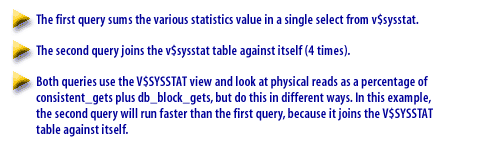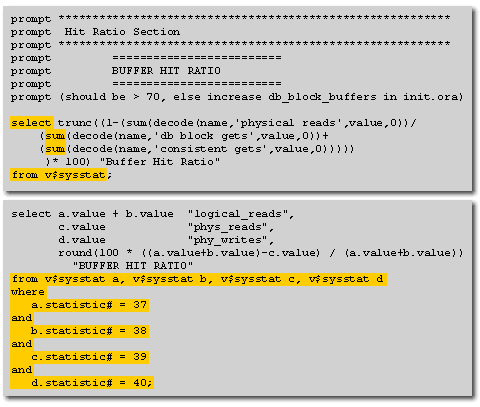Data Dictionary   «Prev

# V\$SYSSTAT is a Dynamic Performance View

How is the Buffer Hit Ratio related to V\$SYSSTAT when conducting performance tuning in Oracle?
V\$SYSSTAT is a dynamic performance view in the Oracle RDBMS that displays system statistics. It includes statistics on user activity, redo log activity, enqueue activity, cache activity, operating system activity, Real Application Clusters (RAC) activity, SQL activity, and debug activity.
The columns in the V\$SYSSTAT view are:
1. STATISTIC#: The number of the statistic.
2. NAME: The name of the statistic.
3. CLASS: A number representing one or more statistics classes. The following class numbers are additive:
1. 1 - User
2. 2 - Redo
3. 4 - Enqueue
4. 8 - Cache
5. 16 - OS
6. 32 - RAC
7. 64 - SQL
8. 128 - Debug
4. VALUE: The value of the statistic.
5. STAT_ID: The identifier of the statistic.
6. CON_ID: The ID of the container to which the data pertains.

## Associated Statistic

To find the name of the statistic associated with each statistic number, you can query the V\$STATNAME view.
The V\$SYSSTAT view is a valuable tool for monitoring the performance of an Oracle database. It can be used to identify potential problems and bottlenecks. Examples:
Here are some examples of how the V\$SYSSTAT view can be used:
1. To monitor user activity, you can query the V\$SYSSTAT view for statistics such as the number of active sessions, the number of logical reads, and the number of physical reads.
2. To monitor redo log activity, you can query the V\$SYSSTAT view for statistics such as the number of redo entries generated, the number of redo blocks written, and the number of redo blocks flushed.
3. To monitor enqueue activity, you can query the V\$SYSSTAT view for statistics such as the number of enqueues granted, the number of enqueues denied, and the number of enqueues in the wait list.
4. To monitor cache activity, you can query the V\$SYSSTAT view for statistics such as the number of cache hits, the number of cache misses, and the number of cache cold misses.
5. To monitor operating system activity, you can query the V\$SYSSTAT view for statistics such as the number of CPU seconds used, the number of physical reads, and the number of physical writes.
6. To monitor RAC activity, you can query the V\$SYSSTAT view for statistics such as the number of RAC transactions, the number of RAC blocks transferred, and the number of RAC deadlocks.
7. To monitor SQL activity, you can query the V\$SYSSTAT view for statistics such as the number of SQL statements executed, the number of SQL rows processed, and the number of SQL errors.
8. To monitor debug activity, you can query the V\$SYSSTAT view for statistics such as the number of debug events, the number of debug messages, and the number of debug errors.

The V\$SYSSTAT view is a powerful tool that can be used to monitor the performance of an Oracle database. By understanding the statistics in this view, you can identify potential problems and bottlenecks, and take steps to improve the performance of your database.

## Computing Data buffer hit ratio in Oracle

Consider the following two queries to compute and display the data buffer hit ratio. This is an excellent example of how the DBA can write the same query in two different ways.Buffer Hit Ratio The first query sums the various statistics value in a single select from V\$sysstat. The second query joins the V\$sysstat table against itself (4 times). Both queries use the V\$sysstat view ad look at physical reads as a percentage of consistent_get plus db_block_gets, but do this in different ways. In this example, the second query will run faster than the first query, because it joins the V\$sysstat table against itself.

## Buffer hit ratioBuffer Hit Ratio The first query sums the various statistics value in a single select from V\$SYSSTAT. The second query joins the V\$SYSSTAT table against itself (4 times).
```PROMPT **************************************
PROMPT  HIT RATIO SECTION
PROMPT **************************************
PROMPT
PROMPT    =========================
PROMPT    BUFFER HIT RATIO
PROMPT    =========================
prompt (should be > 70,
else increase db_block_buffers in init.ora)

select trunc(
(sum(decode(name,'db block gets',value,0))+
(sum(decode(name,'consistent gets',value,0)))))
)* 100) " Buffer hit ratio "
FROM v\$sysstat;
-----------------------------------------------
```

```select a.value + b.value "logical_reads",
d.value            "phy_writes",
round(100*((a.value + b.value)-c.value)/(a.value+b.value))
" buffer hit ratio "
from v\$sysstat a, v\$sysstat b, v\$sysstat c, v\$sysstat d
WHERE
a.statistic# = 37
AND
b.statistic# = 38
AND
c.statistic# = 39
AND
d.statistic# = 40;
```

Both queries use the V\$sysstat 1) view and 2) look at physical reads as a percentage of consistent_gets plus db_block_gets, but do this in different ways. In this example, the second query will run faster than the first query, because it joins the V\$SYSSTAT table against itself.